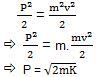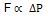# Conservation Of Linear Momentum

## Linear Momentum

We have seen quantities like velocity, acceleration, mass, etc. and know what they signify. Now we will define a quantity known as momentum, which is mathematically represented as:
P = mv
It is the amount of motion a body possesses. As it is a product of mass and velocity, like velocity, it is also a vector quantity. Its unit is Kg m s-1. Now we will relate the kinetic energy with its linear momentum.
P = mv

Squaring both sides and dividing by 2 we get,So if two bodies having different masses have the same kinetic energy than the one with lighter mass has smaller momentum.

## Linear Momentum Formula and Unit

 Linear momentum formula for a particle P=mv Linear momentum formula for ‘n’ no.of particles P=p1+p2+….+pn Unit of linear momentum kg.m.s-1

## How to Calculate the Rate of Change of Momentum?

Newton’s Second law relates force with the rate of change of momentum. According to the law, force is directly proportional to the rate of change in momentum.We will use this to state the law of conservation of momentum. According to this, if the net force acting on the system is zero, then the system’s momentum remains conserved. In other words, the change in momentum of the system is zero. According to the second law, we can see as F = 0, so it will also be zero. Let’s take the following example:
We consider m1 and m2 as our system. So during the collision, the net force on the system is zero, and hence we can conserve the system’s momentum. The equation for momentum will be:

Initial momentum = m1u1 + m2u2

Final momentum = m1v1 + m2v2

So, according to the conservation of momentum,

m1u1 + m2u2 = m1v1 + m2v2

But one thing to take care is that conservation is only true for a system and not one body because if we consider only a single body m1 or m2, then the net force will be acting on it, so we cannot write m1u1 ≠ m1v1 or m2u2 ≠ m2v2.

## Watch the demonstration of conservation of energy and momentum using coins!